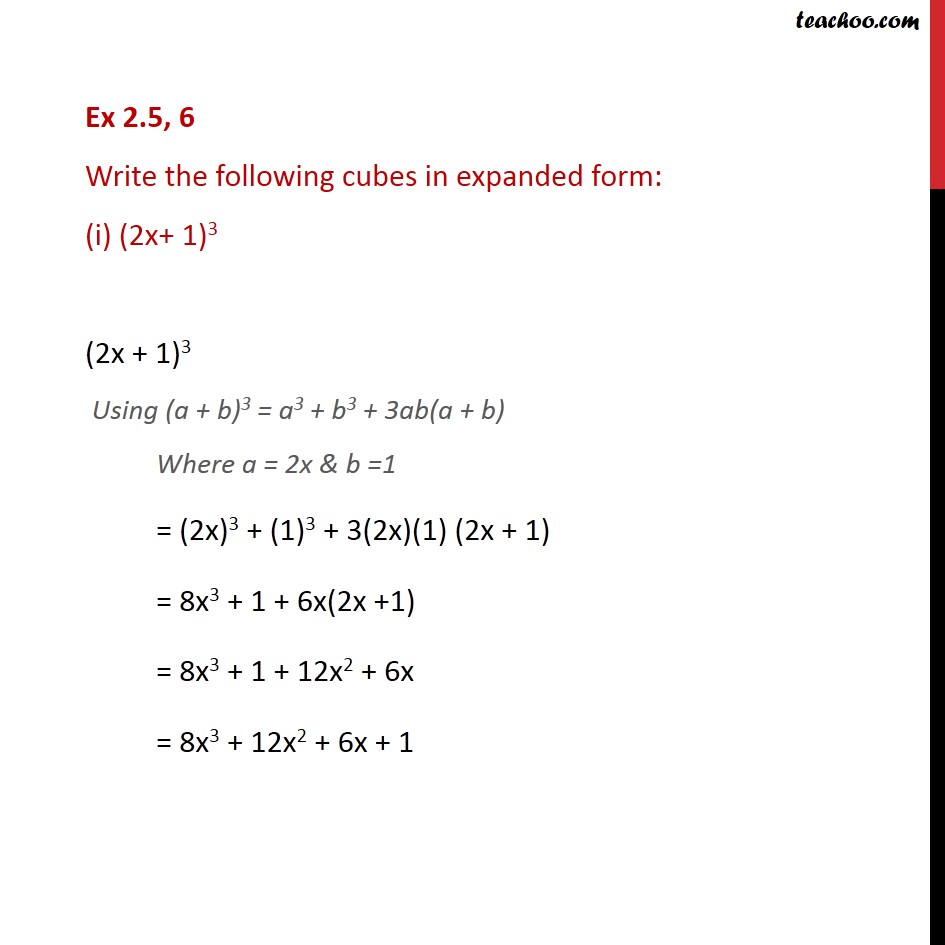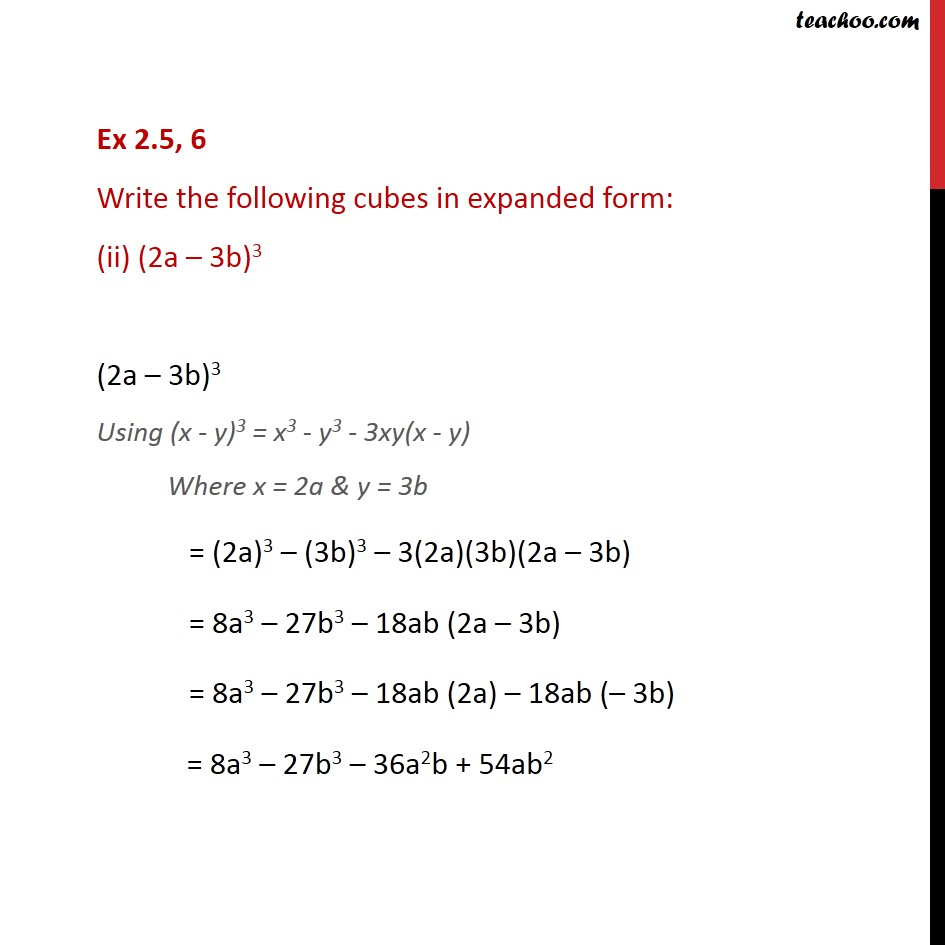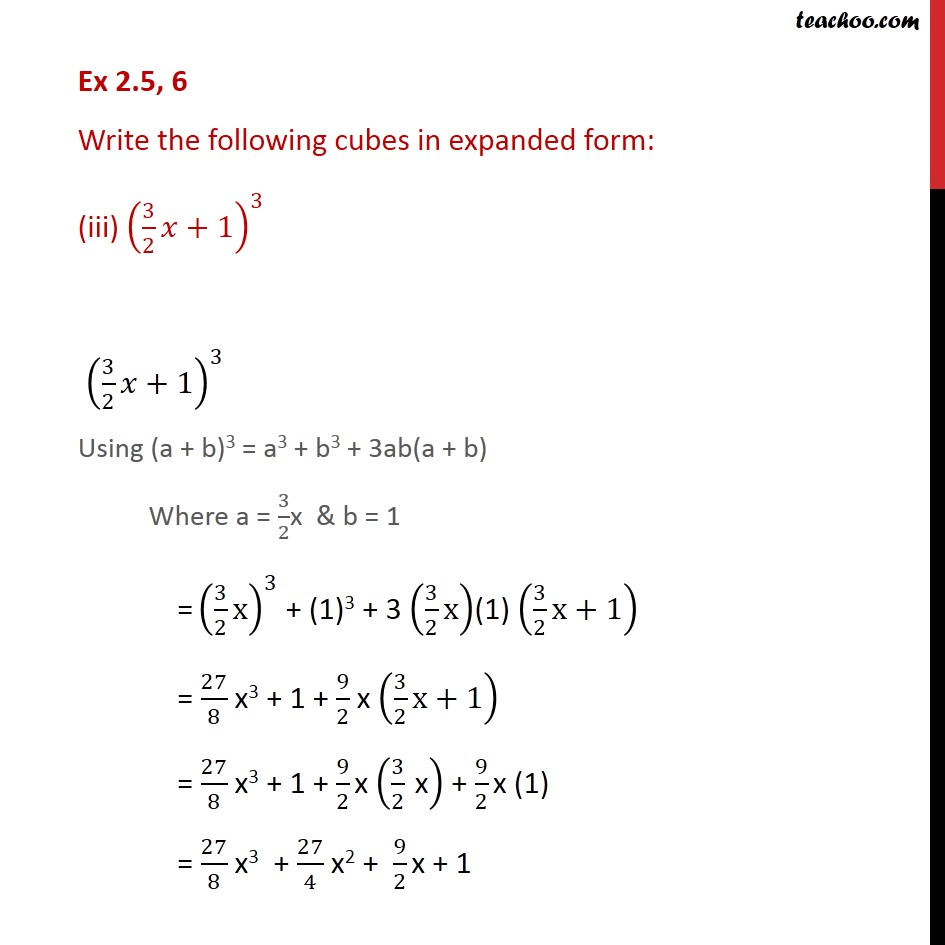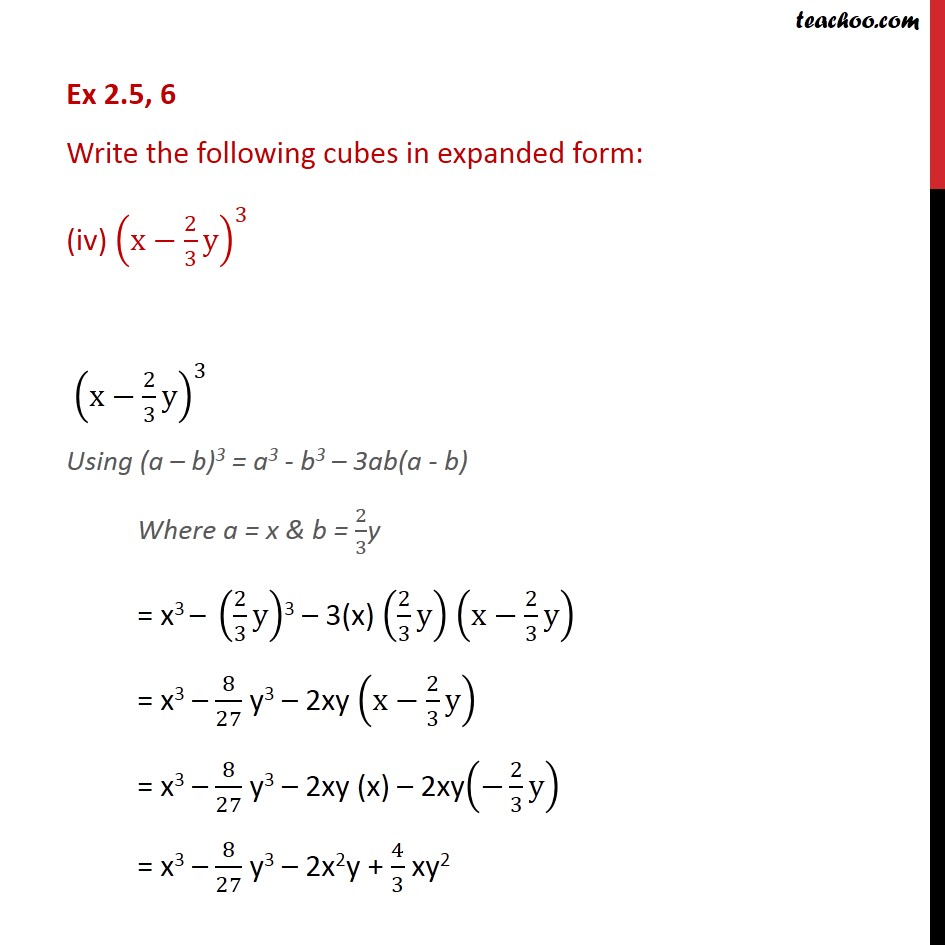1. Chapter 2 Class 9 Polynomials
2. Serial order wise
3. Ex 2.5

Transcript

Ex 2.5, 6 Write the following cubes in expanded form: (i) (2x+ 1)3 (2x + 1)3 Using (a + b)3 = a3 + b3 + 3ab(a + b) Where a = 2x & b =1 = (2x)3 + (1)3 + 3(2x)(1) (2x + 1) = 8x3 + 1 + 6x(2x +1) = 8x3 + 1 + 12x2 + 6x = 8x3 + 12x2 + 6x + 1 Ex 2.5, 6 Write the following cubes in expanded form: (ii) (2a 3b)3 (2a 3b)3 Using (x - y)3 = x3 - y3 - 3xy(x - y) Where x = 2a & y = 3b = (2a)3 (3b)3 3(2a)(3b)(2a 3b) = 8a3 27b3 18ab (2a 3b) = 8a3 27b3 18ab (2a) 18ab ( 3b) = 8a3 27b3 36a2b + 54ab2 Ex 2.5, 6 Write the following cubes in expanded form: (iii) (3/2 +1)^3 (3/2 +1)^3 Using (a + b)3 = a3 + b3 + 3ab(a + b) Where a = 3/2x & b = 1 = (3/2 x)^3 + (1)3 + 3 (3/2 x)(1) (3/2 x+1) = 27/8 x3 + 1 + 9/2 x (3/2 x+1) = 27/8 x3 + 1 + 9/2 x (3/2 " x" ) + 9/2 x (1) = 27/8 x3 + 27/4 x2 + 9/2 x + 1 Ex 2.5, 6 Write the following cubes in expanded form: (iv) (x 2/3 y)^3 (x 2/3 y)^3 Using (a b)3 = a3 - b3 3ab(a - b) Where a = x & b = 2/3y = x3 (2/3 y)3 3(x) (2/3 y) (x 2/3 y) = x3 8/27 y3 2xy (x 2/3 y) = x3 8/27 y3 2xy (x) 2xy( 2/3 y) = x3 8/27 y3 2x2y + 4/3 xy2

Ex 2.5

Chapter 2 Class 9 Polynomials
Serial order wise